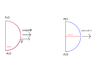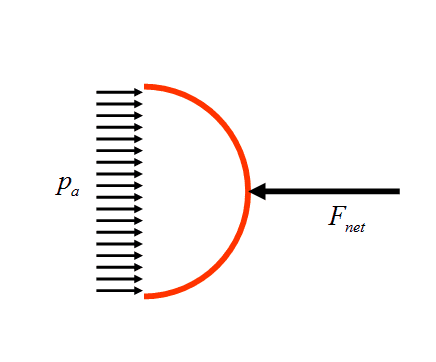# Force and pressure -- Pulling apart two hemispheres with low pressure inside

Elias Waranoi

## Homework Statement

In a lecture demonstration, a professor pulls apart two hemispherical steel shells (diameter D) with ease using their attached handles. She then places them together, pumps out the air to an absolute pressure of p, and hands them to a bodybuilder in the back row to pull apart. (a) If atmospheric pressure is p0, how much force must the bodybuilder exert on each shell?

## Homework Equations

p = F/A
Area of hemisphere A = 2πr2 = πD2/2

## The Attempt at a Solution

So I just took the force pushing on a hemisphere from pressure p0 negative the force pushing out on the the hemisphere from pressure p to get the force needed to pull away. F = (p0 - p)πD2/2.

This answer is not correct apparently, the correct answer is F = (p0 - p)πD2/4. What I'm thinking is that the pulling force required is the x-component of force F but I can't figure how to get that. Also, what direction does F have? If I were to use p = F/A to calculate the force on a sphere what direction would F have? Or is F a scalar? Then how should I get the x-component of scalar?

Gold Member
Hi Elias:

Have you learned about integration yet?

Consider a small area on the surface with area dA and its geometric center at [x,y,z]. What is the force pulling inward from the pressure difference? What is it's x coordinate component? Can you represent the integral over the total area of the x coordinate of the inward force on the hemisphere? It helps to do the math by using spherical coordinates.

But there is a simpler way. Since inward force is proportional to dA everywhere on the surface, the problem reduces to projecting each dA onto a surface normal to the x axis. What you get when you project the entire hemisphere is a circle of diameter D. Since this circle's area is 1/2 of the hemisphere area, that is where the additional factor of 1/2 comes from.

Hope this helps.

Regards,
Buzz

Elias Waranoi
Since inward force is proportional to dA everywhere on the surface, the problem reduces to projecting each dA onto a surface normal to the x axis. What you get when you project the entire hemisphere is a circle of diameter D. Since this circle's area is 1/2 of the hemisphere area, that is where the additional factor of 1/2 comes from.

Wait, how does that work? So if I instead of my hemisphere I had some weird shape that when projected onto a surface makes a circle with diameter D, then regardless of shape the horizontal force on my shape is p * A where A is the area of the circle with diameter D? Is there an intuitive explanation for that?

I have a basic understanding of integrals but I'm really bad at applying them to this stuff. Looking at the hemisphere from the side, if the flat part of the hemisphere goes along the y-axis I thought of taking a slice of the hemisphere then integrate over -π/2 to π/2 with cosine to get the total horizontal force of that slice. Then looking at the hemisphere from above, integrate that slice over -π/2 to π/2 with cosine to get the total horizontal force of all slices. I added an image to show my thoughts. Is this correct? And if so, could you show me how to integrate this?

Edit: Left hemisphere is seen from the side, right is seen from above

#### Attachments

•integr.png
6.4 KB · Views: 614
Gold Member
So if I instead of my hemisphere I had some weird shape that when projected onto a surface makes a circle with diameter D, then regardless of shape the horizontal force on my shape is p * A where A is the area of the circle with diameter D?
Hi Elias.

You quote shows a misunderstanding. This projection requires that the partitioning of the hemisphere into small dA pieces will project along he x-axis to exactly fill up the circle with the projected pieced. Not all surface shapes will do this.It is possible to create a shape so that different dA pieces will project onto the same part of the circle. The spherical shape can be integrated simply to show the same circle area as a result. It will also work for an ellipsoid where he x-axis is one of the symmetry axes.

Regarding the diagram, I am not sure I understand what you are showing. It would help if the diagram shows what happens to a dA piece, and you also provide text to explain what the diagram is intended to show,

Regards,
Buzz

Elias Waranoi
I don't think that I can explain any better. Thanks for the help I've gotten though. I'll have to re-visit this when I get better at integrals.

Gold Member
Pressure on any small element of the shell surface generates a force which is normal to the surface . This force has axial and radial components . The axial components for all the elements sum together to give a net axial force . The radial components all cancel out .

Do the sums and this is the same as saying that pressure effectively acts on the projected area of the shell . ie force is pi R2 x pressure .

•CWatters
Mentor
If you do a free body diagram on a hemisphere (and the air inside it) before the vacuum is applied, and write down the force balance on this combined system, you get $$p_a A_{rim}+p_a \frac{\pi D^2}{4}=F_{net}$$where ##p_a## is atmospheric pressure, ##A_{rim}## is the cross sectional area of the rim, D is the diameter of the cavity, and ##F_{net}## is the net resultant force of the air on the outside of the hemisphere.When you apply vacuum to the inside of the joined hemispheres, the net force of the atmospheric air on the outside of each hemisphere doesn't change. So, it is still ##p_a \left(A_{rim}+\frac{\pi D^2}{4}\right)##

Hi Elias:

Have you learned about integration yet?

Consider a small area on the surface with area dA and its geometric center at [x,y,z]. What is the force pulling inward from the pressure difference? What is it's x coordinate component? Can you represent the integral over the total area of the x coordinate of the inward force on the hemisphere? It helps to do the math by using spherical coordinates.

But there is a simpler way. Since inward force is proportional to dA everywhere on the surface, the problem reduces to projecting each dA onto a surface normal to the x axis. What you get when you project the entire hemisphere is a circle of diameter D. Since this circle's area is 1/2 of the hemisphere area, that is where the additional factor of 1/2 comes from.

Hope this helps.

Regards,
Buzz
Kind of right. The projection on to a surface normal to the x-axis is a circle. The formula for area of a circle is pi r squared or pi * (D/2)^2. This is equivalent to pi * D^2/4. So the question is not, 'have you learned about integration yet?', it's 'have you learned about algebra yet?' There is no additional factor of 1/2; it's what happens when you square a two in the denominator.

•Tom.G